# Four Points Puzzle

Take four distinct points and plot them on a 2D plane. Then connect each pair of points with an edge. There will always be 4C2 = 6 edges that will be created, no matter the geometry. Now, measure the length of each of these edges. The chances are there a lot of different lengths. The challenge for today is to come up with a geometric arrangement of the points so that there are only two distinct edge lengths.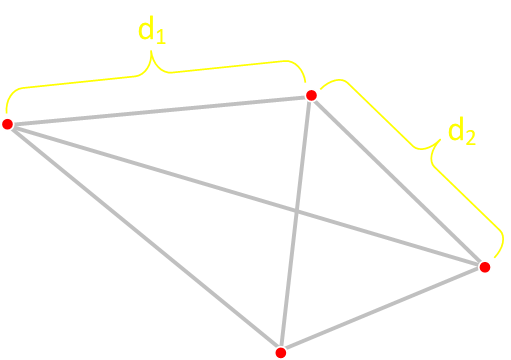This is a classic geometry puzzle. I can’t find the exact origin, but I believe it dates back to the Martin Gardner era. Martin Gardner is a real hero of mine, I wish I’d had the chance to meet him; he inspired me from an early age.
What are the layouts for four points such that there are only two distinct distances between any pair of them?
With a little thinking, in a short time, you will probably come up with a solution. The most common first answer, from the non-scientific survey of a few friends, seems to be a square arrangement. In this configuration there are four ‘short’ edges for the perimeter of the square, and the two ‘long’ diagonals.Ignoring trivial reflections and rotations, how many distinct classes of solution can you find to this puzzle? If you are feeling brave, how would you go about proving that there are no more?
Ignoring trivial reflections and rotations, how many distinct classes of solution can you find to this puzzle?

## Thinking

Thinking about this, it’s easy to see that there needs to be at least two distinct edge lengths. There is no way to arrange four points (on a plane) that are equidistant from each other (one distinct length). The first three points would need to be arranged in an equilateral triangle, and the only way to place the fourth point would be to move it out of the plane to make the vertices of a tetrahedron.
Similarly, with a bit of thinking, we can determine that no three points can be colinear. If we place two points, then the third point would need to be the same distance away along the line to ensure there were just two distinct lengths. With this done, there is no way to place a fourth point that does not break the criterion of having two distinct lengths.Triangles are very important in this puzzle, and with some logic we can show that each edge is in exactly two triangles, and that at least two ‘short’ edges share a vertex.
We can’t have zero short edges because of the tetrahedron issue described above (Similarly, we can’t have zero long edges). We also can’t have just one short edge because the other five (long) edges would need to be arranged as two equilateral triangles sharing a common edge, and so the missing diagonal edge would be longer.
If there are two short edges not sharing a vertex, they would have to bisect perpendicular and that doesn’t work, so, if there are exactly two short edges, these two edges must share a vertex.

## Give it a go?

Here's a short app to allow you to experiment with trying to find solutions. Use your mouse, or finger, to drag the vertices around. If you find a solution, it will be indicated, and the edges will turn bold.
To aid you, at the bottom, is a table showing the (rounded to the nearest integer) edge lengths, and an indication of how many distinct edges there currently are. Want more of a challenge? Turn this table off.
By default, the app starts in low accuracy mode. In this setting, you don't need to be pixel perfect and you can find a solution with a little 'slop' in each vertex. This should also help with small displays if you are experimenting on a mobile device. There are three levels of accuracy, with the highest requiring pixel accuracy. If you are having difficulty locking onto a solution, you can start at low, get a hit, then switch up the accuracy and refine it.
Finally, there is a colour mode option. This almost makes it too easy! If you switch to colour mode, each distinct length gets its own colour. This quickly allows you to know what is matching and what needs to be tweaked.

## Solutions

There are, in fact, six distinct classes of solution. How many did you get? I’ll enumerate them below, then show my attempt at proving these are the only ones. I’ll present these in the order of ‘difficulty’ that (in my non-scientific, and small sample) my friends uncovered them.## SquareThis is the solution that most people come up with first; Four shorter edges with the cross-braced diagonals. The longer edges are √2 times the length of the short edges. Simple!
Short Edges: 4    Long Edges: 2

## RhombusIf we 'pull' two opposite corners of the square apart, we can make a rhombus. This, initially, breaks things as the two diagonals will have different lengths (making four distinct lengths), but if we keep pulling there is a critical point at which the shorter of the diagonals matches the length of the sides. When this happens, we're back to two distinct lengths and have another solution. If you look closely you will see that this rhombus is made up of two equilateral triangles that share a common edge, with a long cross brace between the vertices that do not share the common edge.
Short Edges: 5    Long Edges: 1

## Delta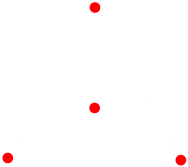The next solution is to start off with an equilateral triangle made of long edges, then place the last vertex at the centroid of this triangle. As the centroid is equidistant from each of the corners, these short edges are all the same length.
Short Edges: 3    Long Edges: 3

## KiteHere we start with an equilateral triangle made up from longer edges then descend another edge, bisecting one corner. Two short lengths are used to then connect the two vertices at the base.
Short Edges: 2    Long Edges: 4

## Dart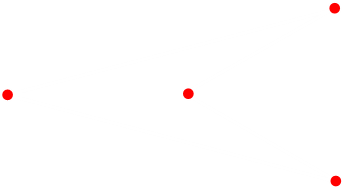This dart configuration looks a little like a Star Trek logo! We start with an equilateral triangle made from shorter edges, then place the final vertex a distance out from one of the triangle vertices the same short distance. The longer edges form an isosceles triangle with the base of the shorter edge.
Short Edges: 4    Long Edges: 2

## TrapezoidThis final configuration is usually the last solution found. It consists of three short edges and three long edges to form a trapezoid. One way to think about this configuration is to imagine this as a regular pentagram from which one vertex has been removed. On a regular pentagon, the perimeter lengths are all the same length (here the short edges), as are the internal diagonals which are the long lengths. (You can also think of this as two isoselese that have 'fallen' into each other to share a common long edge).
Short Edges: 3    Long Edges: 3

## Play with the solutions

Here is a second version of the app that shows the solutions. Use the buttons at the bottom to load each of the six solutions and experiment.

## Proof

OK, how do we go about proving there are no more solutions than these six? I'm sure there are some elegant logical proofs, but here is my attempt by using a little brute force and enumerating out all possible ways, and then eliminating the impossible. In true Sherlock Holmes* style, whatever is left has to be a solution.

* "When you have eliminated the impossible, whatever remains, however improbable, must be the truth."
Sherlock Holmes - The Sign of the Four, by Sir Arthur Ignatius Conan Doyle
(I think it's fitting that this quote comes from the book of that title!)

OK here goes:
Let's label the four points: A B C D.
Without loss of generality, we'll place point A at the origin (0,0) of a cartesian grid. Next, we'll place point B at the coordinates (1,0). We can do this as we don't care about scaling factors or rotations.This leaves two points outstanding: C and D. They could be anywhere, but, we have constraints. Because, for a solution, there needs to be only two distinct lengths, the distances from AC, AD, CD, BC, BD are quanatized to one of two values: Either 1 (the distance from AB), or another, yet to be calculated, value (that could be greater or less then 1 depending on if AB is the 'short' or 'long' edge).
Another way to think about this is that we know, for instance, that C needs to be somewhere on a circle centered on the origin and with either a radius of 1 or another radius value we'll call L. Similarly, point D will be on a circle centered on the original with one of these two radii …What we have is a set of simultaneous equations that we can solve to determine the coordinates of C and D. If we use (xC, yC) to represent the coordinates of C, and (xD, yD) to represent the coordinates of D, and applying Pythagoras, we can derive six equations: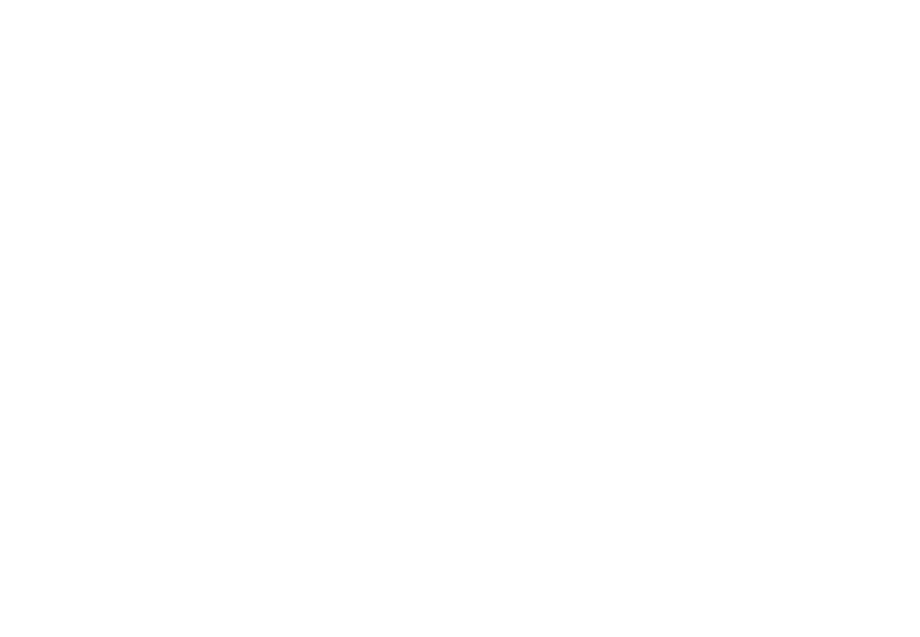## Enumeration

Here is where the brute force comes in. We have defined AB=1. Each of these other lengths is either the same as AB, or another value, which we're calling L. It's a boolean choice; each of these other lengths is either the same as AB, or not. As there are 5 lengths, there are 25=32 possible combinations. Think of these combinations like binary numbers 00000-11111 (0-31 in decimal), where a digit of 1 represents that length being the same as AB, and the digit 0 representing that length being L, with each binary digit position representing one of the five lengths we are concerned about.
We can go a little further, as we don't care about scaling, and say that the length of AB is the longer of the two edges so that L<1. In this way we know that some solutions, such as 11111 are impossible as all lengths would be the same - the tetrahedron issue. We also know that there can't be just one short edge, so there can be no solution that has a single '0' digit in the table, but as we will see below, this comes out in the math anyway.
# Binary # Binary # Binary # Binary
0 → 00000 8 → 01000 16 → 10000 24 → 11000
1 → 00001 9 → 01001 17 → 10001 25 → 11001
2 → 00010 10 → 01010 18 → 10010 26 → 11010
3 → 00011 11 → 01011 19 → 10011 27 → 11011
4 → 00100 12 → 01100 20 → 10100 28 → 11100
5 → 00101 13 → 01101 21 → 10101 29 → 11101
6 → 00110 14 → 01110 22 → 10110 30 → 11110
7 → 00111 15 → 01111 23 → 10111 31 → 11111

## Bring on the Simultaneous Equations!

Using the digits of the binary number (left to right), to represent the lengths, AC, AD, BC, BD, CD respectively, we can create five simultaneous equations for each possible combination of short and long legnths. Where the digit is a "1" we equate this length to 1. Where the digit is '0' we equate this length to L. All these equations are shown in nauseating detail below:
``````
0. xc2 + yc2 = L2, xd2 + yd2 = L2, (xc-1)2 + yc2 = L2, (xd-1)2 + yd2 = L2, (xc-xd)2 + (yc-yd)2 = L2
1. xc2 + yc2 = L2, xd2 + yd2 = L2, (xc-1)2 + yc2 = L2, (xd-1)2 + yd2 = L2, (xc-xd)2 + (yc-yd)2 = 1
2. xc2 + yc2 = L2, xd2 + yd2 = L2, (xc-1)2 + yc2 = L2, (xd-1)2 + yd2 = 1, (xc-xd)2 + (yc-yd)2 = L2
3. xc2 + yc2 = L2, xd2 + yd2 = L2, (xc-1)2 + yc2 = L2, (xd-1)2 + yd2 = 1, (xc-xd)2 + (yc-yd)2 = 1
4. xc2 + yc2 = L2, xd2 + yd2 = L2, (xc-1)2 + yc2 = 1, (xd-1)2 + yd2 = L2, (xc-xd)2 + (yc-yd)2 = L2
5. xc2 + yc2 = L2, xd2 + yd2 = L2, (xc-1)2 + yc2 = 1, (xd-1)2 + yd2 = L2, (xc-xd)2 + (yc-yd)2 = 1
6. xc2 + yc2 = L2, xd2 + yd2 = L2, (xc-1)2 + yc2 = 1, (xd-1)2 + yd2 = 1, (xc-xd)2 + (yc-yd)2 = L2
7. xc2 + yc2 = L2, xd2 + yd2 = L2, (xc-1)2 + yc2 = 1, (xd-1)2 + yd2 = 1, (xc-xd)2 + (yc-yd)2 = 1
8. xc2 + yc2 = L2, xd2 + yd2 = 1, (xc-1)2 + yc2 = L2, (xd-1)2 + yd2 = L2, (xc-xd)2 + (yc-yd)2 = L2
9. xc2 + yc2 = L2, xd2 + yd2 = 1, (xc-1)2 + yc2 = L2, (xd-1)2 + yd2 = L2, (xc-xd)2 + (yc-yd)2 = 1
10. xc2 + yc2 = L2, xd2 + yd2 = 1, (xc-1)2 + yc2 = L2, (xd-1)2 + yd2 = 1, (xc-xd)2 + (yc-yd)2 = L2
11. xc2 + yc2 = L2, xd2 + yd2 = 1, (xc-1)2 + yc2 = L2, (xd-1)2 + yd2 = 1, (xc-xd)2 + (yc-yd)2 = 1
12. xc2 + yc2 = L2, xd2 + yd2 = 1, (xc-1)2 + yc2 = 1, (xd-1)2 + yd2 = L2, (xc-xd)2 + (yc-yd)2 = L2
13. xc2 + yc2 = L2, xd2 + yd2 = 1, (xc-1)2 + yc2 = 1, (xd-1)2 + yd2 = L2, (xc-xd)2 + (yc-yd)2 = 1
14. xc2 + yc2 = L2, xd2 + yd2 = 1, (xc-1)2 + yc2 = 1, (xd-1)2 + yd2 = 1, (xc-xd)2 + (yc-yd)2 = L2
15. xc2 + yc2 = L2, xd2 + yd2 = 1, (xc-1)2 + yc2 = 1, (xd-1)2 + yd2 = 1, (xc-xd)2 + (yc-yd)2 = 1
16. xc2 + yc2 = 1, xd2 + yd2 = L2, (xc-1)2 + yc2 = L2, (xd-1)2 + yd2 = L2, (xc-xd)2 + (yc-yd)2 = L2
17. xc2 + yc2 = 1, xd2 + yd2 = L2, (xc-1)2 + yc2 = L2, (xd-1)2 + yd2 = L2, (xc-xd)2 + (yc-yd)2 = 1
18. xc2 + yc2 = 1, xd2 + yd2 = L2, (xc-1)2 + yc2 = L2, (xd-1)2 + yd2 = 1, (xc-xd)2 + (yc-yd)2 = L2
19. xc2 + yc2 = 1, xd2 + yd2 = L2, (xc-1)2 + yc2 = L2, (xd-1)2 + yd2 = 1, (xc-xd)2 + (yc-yd)2 = 1
20. xc2 + yc2 = 1, xd2 + yd2 = L2, (xc-1)2 + yc2 = 1, (xd-1)2 + yd2 = L2, (xc-xd)2 + (yc-yd)2 = L2
21. xc2 + yc2 = 1, xd2 + yd2 = L2, (xc-1)2 + yc2 = 1, (xd-1)2 + yd2 = L2, (xc-xd)2 + (yc-yd)2 = 1
22. xc2 + yc2 = 1, xd2 + yd2 = L2, (xc-1)2 + yc2 = 1, (xd-1)2 + yd2 = 1, (xc-xd)2 + (yc-yd)2 = L2
23. xc2 + yc2 = 1, xd2 + yd2 = L2, (xc-1)2 + yc2 = 1, (xd-1)2 + yd2 = 1, (xc-xd)2 + (yc-yd)2 = 1
24. xc2 + yc2 = 1, xd2 + yd2 = 1, (xc-1)2 + yc2 = L2, (xd-1)2 + yd2 = L2, (xc-xd)2 + (yc-yd)2 = L2
25. xc2 + yc2 = 1, xd2 + yd2 = 1, (xc-1)2 + yc2 = L2, (xd-1)2 + yd2 = L2, (xc-xd)2 + (yc-yd)2 = 1
26. xc2 + yc2 = 1, xd2 + yd2 = 1, (xc-1)2 + yc2 = L2, (xd-1)2 + yd2 = 1, (xc-xd)2 + (yc-yd)2 = L2
27. xc2 + yc2 = 1, xd2 + yd2 = 1, (xc-1)2 + yc2 = L2, (xd-1)2 + yd2 = 1, (xc-xd)2 + (yc-yd)2 = 1
28. xc2 + yc2 = 1, xd2 + yd2 = 1, (xc-1)2 + yc2 = 1, (xd-1)2 + yd2 = L2, (xc-xd)2 + (yc-yd)2 = L2
29. xc2 + yc2 = 1, xd2 + yd2 = 1, (xc-1)2 + yc2 = 1, (xd-1)2 + yd2 = L2, (xc-xd)2 + (yc-yd)2 = 1
30. xc2 + yc2 = 1, xd2 + yd2 = 1, (xc-1)2 + yc2 = 1, (xd-1)2 + yd2 = 1, (xc-xd)2 + (yc-yd)2 = L2
31. xc2 + yc2 = 1, xd2 + yd2 = 1, (xc-1)2 + yc2 = 1, (xd-1)2 + yd2 = 1, (xc-xd)2 + (yc-yd)2 = 1

```
```
Each line contains five equations, and five unknowns (with the exception of the last potential solution, which only has four unknowns, but we already know this will not make a solution because of the tetrahedron issue.
The five unknowns are: xC, yC, xD, yD, and L (the shorter length).

## Solving

I'll show solving one example then, in the interest of space, cut to the a summary showing the results of all the solutions. Let's look at the top row, potential solution #00000.
We start with the five equations for the all the length edges (except AB, which we've defined as 1), then do the necessary substitutions. As this is potential solution #00000 all the other edge lengths are set to L (if there was a '1' in any position we would set the result of that equation to be 1, instead of L).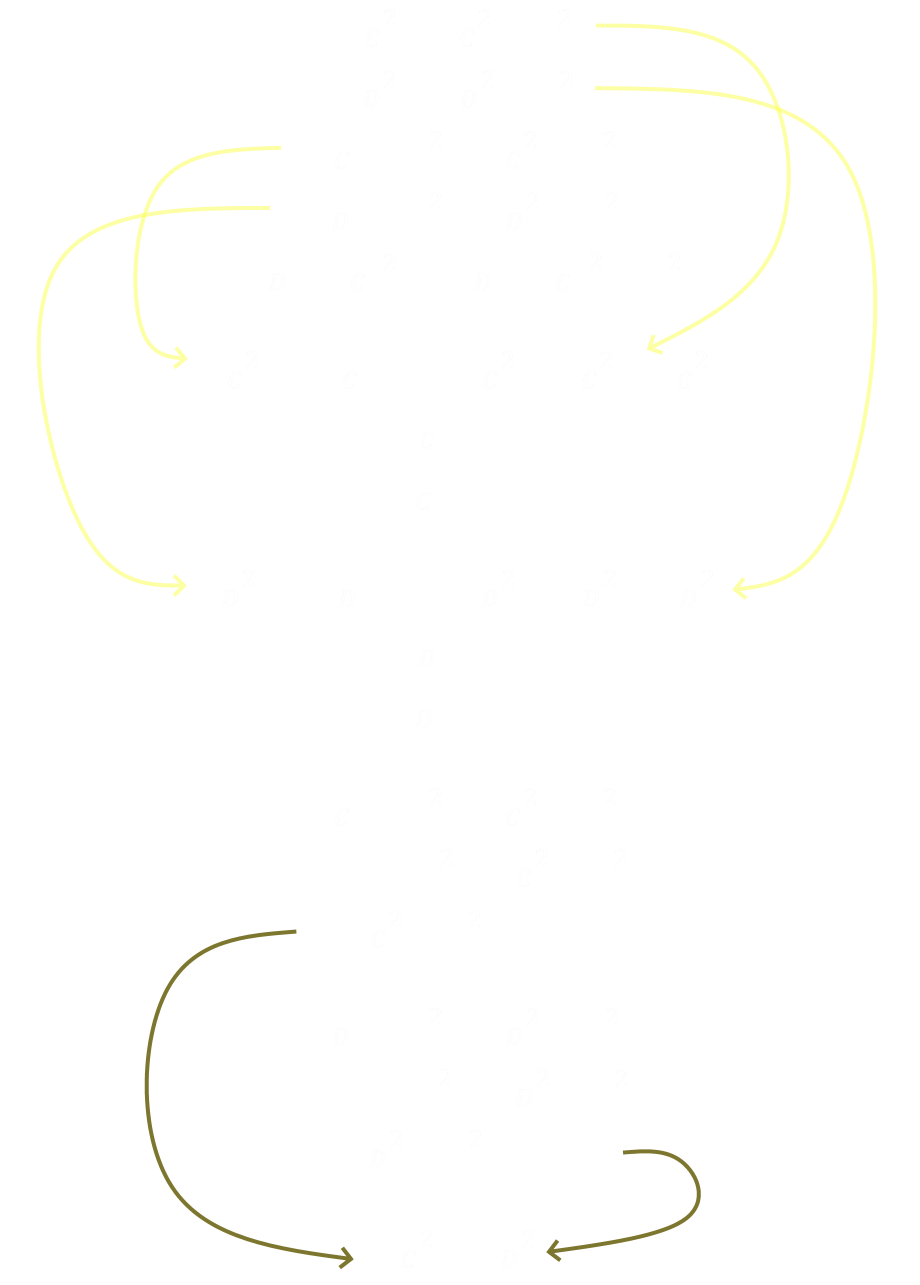We now have the x-coordinates for points C and D. Next we can substitute to get the value for the second edge length, which turns out to be L=1/√3Finally, with substitutions into the top two equations, we can determine the values for the y-coordinates of C and D. With the squared term being in there, there are two solutions ± so we can say that point C is in the first quadrant, and thus we have all the answers:
xC = 1/2   yC = 1/(2√3)   xD = 1/2   yD = -1/(2√3)   L=1/√3
Plotting these out we see that #00000 gives the Rhombus solution!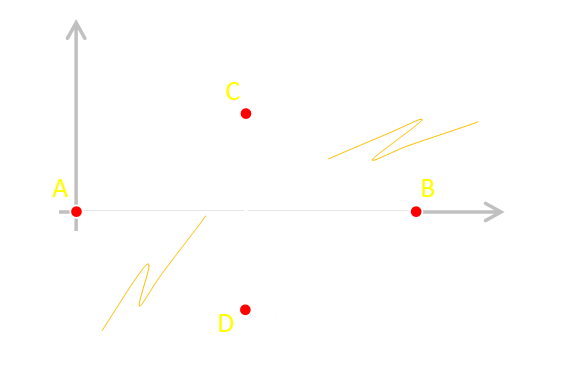## All Solutions

Here are the results of solving each set of simultaneous equations. Note there are some redundant reflections and rotations in there, but the important point is that there are no new classes of solution. We've enumarated through all possible permutations of long and short, and the only solutions found are the six we described above. Result!
# Binary Type XC YC XD YD L
0 00000 Rhombus 1/2 1/(2√3) 1/2 -1/(2√3) 1/√3
1 00001 Square 1/2 1/2 1/2 1/2 1/√2
2 00010 Dart 1/2 (2-√3)/2 (2-√3)/2 1/2 (√3 -1)/√2
3 00011 Trapezoid 1/2 (√(5-2√5))/2 1-(1+√5)/4 -(√((5-√5)/2))/2 (√5 -1)/2
4 00100 Dart (2-√3)/2 1/2 1/2 (2-√3)/2 (√3 -1)/√2
5 00101 Trapezoid 1-(1+√5)/4 (√((5-√5)/2))/2 1/2 -(√(5-2√5))/2 (√5 -1)/2
6 00110 *NO SOLUTION* - - - - -
7 00111 Kite (2-√3)/2 1/2 (2-√3)/2 -1/2 (√3 -1)/√2
8 01000 Dart 1/2 (2-√3)/2 √3/2 1/2 (√3 -1)/√2
9 01001 Trapezoid 1/2 (√(5-2√5))/2 (1+√5)/4 -(√(5-2√5))/2 (√5 -1)/2
10 01010 Delta 1/2 1/(2√3) 1/2 √3/2 1/√3
11 01011 Kite 1/2 (2-√3)/2 1/2 -√3/2 (√3 -1)/√2
12 01100 Trapezoid 1-(1+√5)/4 (√(5-2√5))/2 (1+√5)/4 (√(5-2√5))/2 (√5 -1)/2
13 01101 *NO SOLUTION* - - - - -
14 01110 Kite (2-√3)/2 1/2 1/2 √3/2 (√3 -1)/√2
15 01111 *NO SOLUTION* - - - - -
16 10000 Dart √3/2 1/2 1/2 (2-√3)/2 (√3 -1)/√2
17 10001 Trapezoid (1+√5)/4 (√(5-2√5))/2 1/2 -(√(5-2√5))/2 (√5 -1)/2
18 10010 Trapezoid (1+√5)/4 (√(5-2√5))/2 1-(1+√5)/4 (√(5-2√5))/2 (√5 -1)/2
19 10011 *NO SOLUTION* - - - - -
20 10100 Delta .5 √3/2 .5 1/(2√3) 1/√3
21 10101 Kite 1/2 √3/2 1/2 -(2-√3)/2 (√3 -1)/√2
22 10110 Kite 1/2 √3/2 (2-√3)/2 1/2 (√3 -1)/√2
23 10111 *NO SOLUTION* - - - - -
24 11000 *NO SOLUTION* - - - - -
25 11001 Kite √3/2 1/2 √3/2 -1/2 (√3 -1)/√2
26 11010 Kite √3/2 1/2 1/2 √3/2 (√3 -1)/√2
27 11011 *NO SOLUTION* - - - - -
28 11100 Kite 1/2 √3/2 √3/2 1/2 (√3 -1)/√2
29 11101 *NO SOLUTION* - - - - -
30 11110 *NO SOLUTION* - - - - -
31 11111 *NO SOLUTION* - - - - -
We've shown, using brute force, that there can be no additional classes of solution.